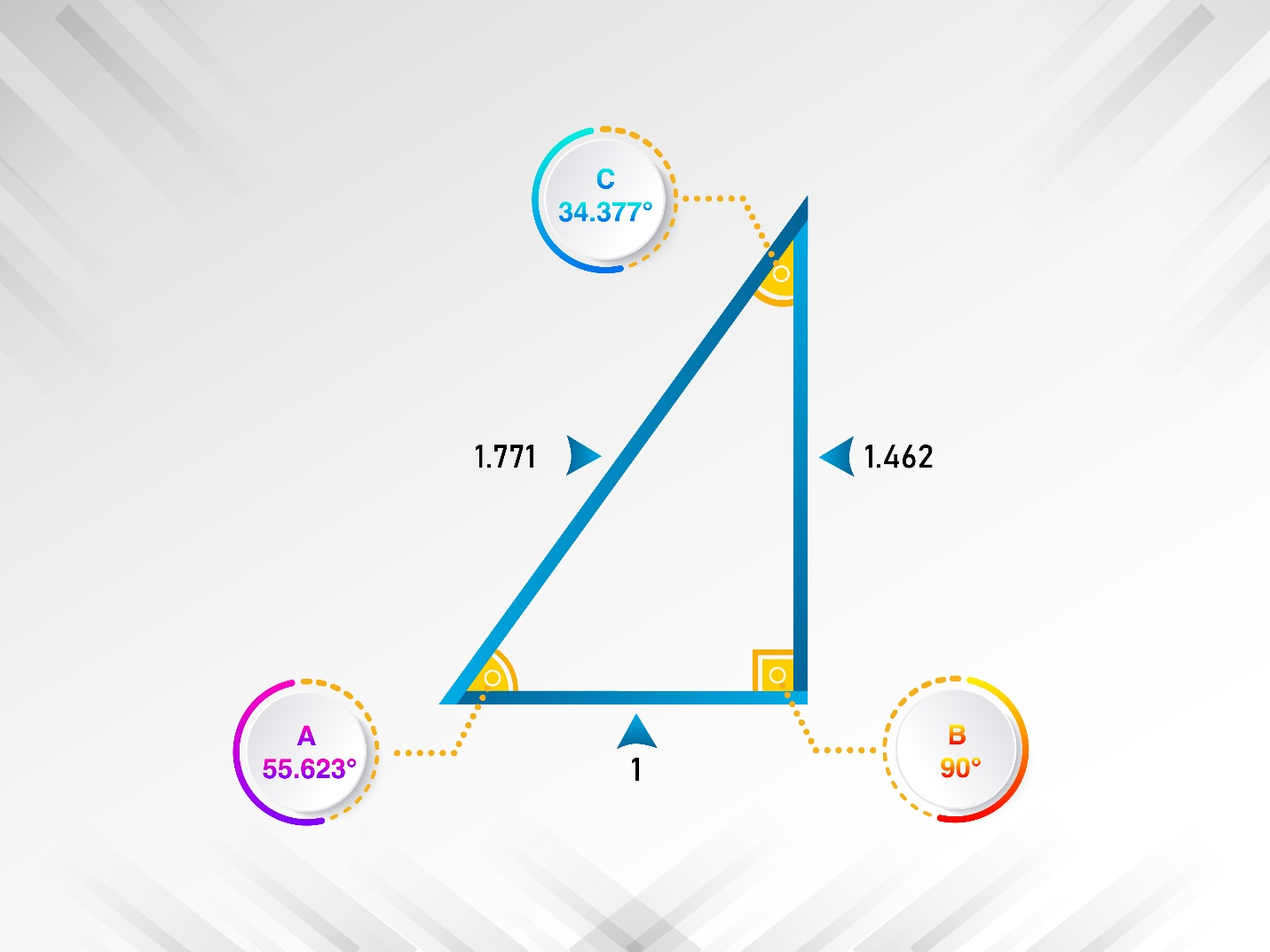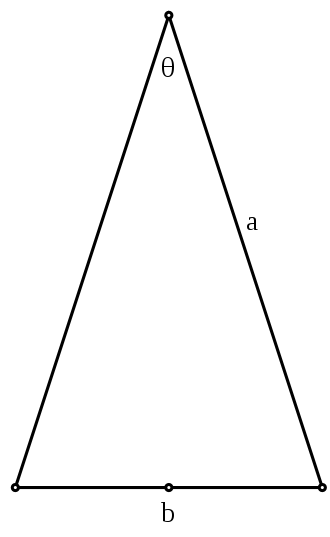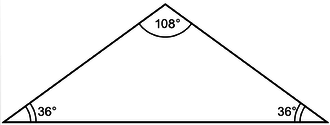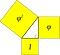# A New Golden Triangle

By Jim FonsecaFigure 1: The Golden Right Triangle

There are several well-known triangles, colloquially called golden triangles, which incorporate some aspect of the mathematical ratio know as phi, 1.61803…, symbolized by the Greek letter Φ. The triangles are called ‘golden’ because that mathematical constant is referred to as the Golden Ratio. That ratio comes about from the idea of cutting a line into two pieces such that the ratio of the two pieces to each other equals the ratio of the larger piece to the original whole piece.

An example helps. So think of the Golden Ratio this way. You have a wooden yardstick, 36 inches. Measure off 22¼ inches and cut it. The remaining short piece will be 13¾ inches. Now do the math: 36/22.25 is 1.618. But 22.25/13.75 is also 1.618. That’s the Golden Ratio. The Golden Ratio is the only way you can cut the stick to satisfy those initial conditions. That’s what makes it so special. And like π, the value of Φ, 1.61803…., is an endless non-repeating fraction.

Previous studies of Golden Triangles have always started with the sides of triangles, probably because we learn about Φ in terms of length and pieces, like in the example I just gave. But in a recent article on ResearchGate, I proposed a new approach to looking for relationships in triangles by starting with the angles of a triangle. After all, while mathematicians may talk about trilaterals, in everyday conversation everyone else speaks of triangles. You can see the full research article including references here

https://www.researchgate.net/publication/350326954_Introducing_A_New_Golden_Triangle_Golden_Quadrilaterals_a_Golden_Irregular_Pentagon_and_Other_Phigons

We know the sum of the angles of a triangle equals 180°. Once we know of the Φ relationship and its importance in many aspects of geometry, it seems perfectly logical to ask the simple question: “If the three angles of a triangle were in phi proportion to each other what would these angles be?”

Based on how we calculate phi the formula to answer this question becomes

This simplifies to

When we solve the formula, x is precisely 90°. The other two angles as shown in Figure 1 are approximately 55.623° (angle A) and 34.377° (angle C). If we do the math for the angles, B/A and A/C each gives us 1.61803, the Φ ratio. I would argue that this triangle is another one deserving of classification as a “golden triangle.” We can call it the Fonseca Golden Triangle. (Just kidding!) Seriously, an appropriate name might be the Golden Right Triangle, shown in Figure 1, above.

The Golden Right Triangle above is different from other triangles also called Golden Triangles. The one most commonly referred to as the Golden Triangle, shown in Figure 2, is an isosceles triangle in which the two longer sides are in Φ ratio to the base. Its angles are 72°, 72° and 36°.Figure 2: The traditional Golden Triangle (from Wikipedia)

Another such triangle is called the Golden Gnomon, Figure 3. It’s also an isosceles triangle in which the ratio of the equal sides to the base is the reciprocal of the Φ ratio. Its angles are 108°, 36° and 36°.Figure 3: The Golden Gnomon (from Wikipedia)

The Kepler triangle is another type of golden triangle, Figure 4. The Kepler triangle is a right triangle and the ratio of its sides to the base is .61803, the reciprocal of Φ. The angles of the Kepler are 90°, and approximately 51.8° and 38.2°. The areas of the squares formed by the edges of this triangle are also in a geometric progression of the Φ ratio: 1, Φ and Φ2. The Kepler triangle is a right triangle like the one in figure 1, but with different second and third angles.Figure 4: The Kepler Triangle (from Wikipedia)

All of the three traditional golden triangles above are determined in one way or another by the length of sides. This is due to the traditional way we learn about Φ, by the division of a line into parts as we saw above. Our focus is always on lines and length, so we approach triangles based on their sides.

And yet we know that a triangle with three sides in Φ proportion is a real-world impossibility (at least in plane geometry) because in order to create a triangle, any two sides must exceed the length of the third side. In the Φ ratio discovered through dividing a line, such a feat is impossible because side lengths in Φ proportion, such as our yardstick and its 22¼ and 13¾ inch pieces, do not meet the basic criterion to form a triangle — they’re too short! This focus on length, the laterals of the triangle, also tends to lead us to treat whatever we learn about the angles of the triangles as somewhat incidental. The new Golden Triangle I described above focuses on the angles.

For those curious about the sides of the Golden Right Triangle in Figure 1, the ratio of the hypotenuse to the longer side is 1.211. I did not find any Φ-related ratios in the figure’s sides. But there may be other insights to be found by comparing this Golden Right Triangle to the traditional Golden Triangle, the Golden Gnomon and the Kepler triangle by comparing its subtriangles formed by mid-points of sides and mid-points of angles.

That’s it for now on triangles but follow my posts on Medium.com if you want to see shortly my posts about applying this Φ formula to Golden Quadrilaterals and a Golden Irregular Pentagon.

I’m James W. Fonseca, a retired geography professor. I’ve done some other work with the Φ ratio and the Fibonacci series as related to urban geography as shown on ResearchGate. My vita is here https://jimwfonseca.squarespace.com/vita

Retired as Dean and geography professor at Ohio University Zanesville. I spend time in Florida, Maine, Chicago and Newport, Rhode Island.

## More from Jim Fonseca

Retired as Dean and geography professor at Ohio University Zanesville. I spend time in Florida, Maine, Chicago and Newport, Rhode Island.